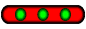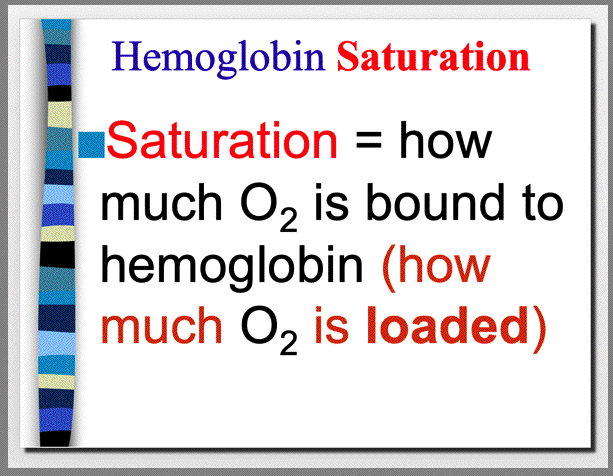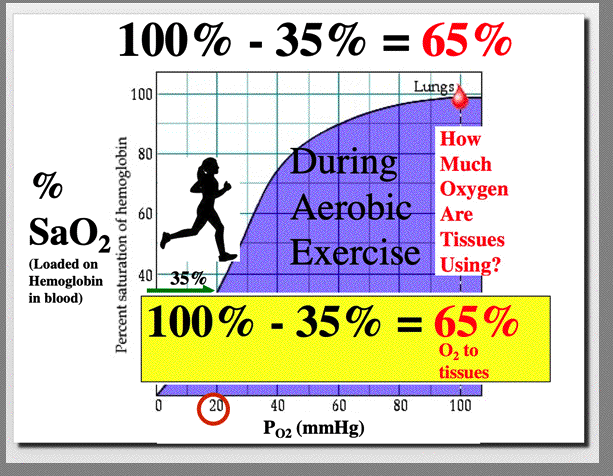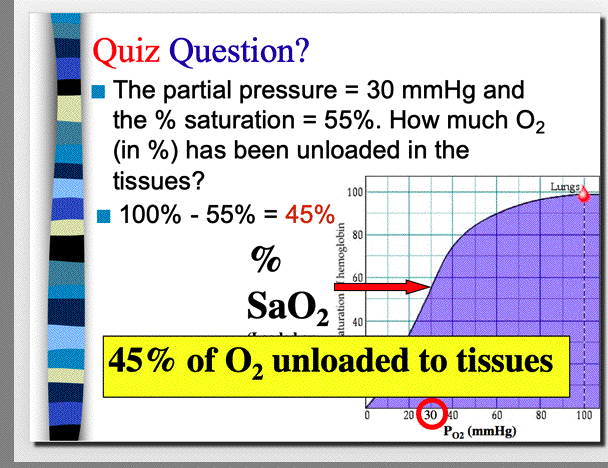## Understanding the Oxygen-hemoglobin Dissociaion Curve

 Ex Phys HomeLet's interpret this Figure. On the 'X' axis is Partial Pressure of oxygen. In a resting state, the Partial Pressure of oxygen in the blood is about 40mmHg. On the 'Y' axis is the Percent Saturation of Oxygen that is loaded on the Hemoglobin in the blood. We see that the Persent Saturation is 75%. Therefore, to answer the question, 'How Much Oxygen Are the Tissues Using' we do a simple calculation: 100% -75% = 25%. The tissues are using 25% of the oxygen in the blood while in a resting state.Let's interpret this Figure. On the 'X' axis is Partial Pressure of oxygen. In an exercise condition, the Partial Pressure of oxygen in the blood is about 20mmHg. On the 'Y' axis is the Percent Saturation of Oxygen that is loaded on the Hemoglobin in the blood. We see that the Persent Saturation is 35%. Therefore, to answer the question, 'How Much Oxygen Are the Tissues Using' we do a simple calculation: 100% -35% = 65%. The tissues are using 65% of the oxygen in the blood while in an exercise condition.See if you can answer this Quiz Question!In completing this quiz question, even thought I give you the Partial Pressure of Oxygen, note that you do not need that number for the calculation. In the word problem I tell you the percent saturation is 55%. That tells us that 55% of the oxygen in blood is loaded on the hemoglobin. Then, the question asks how much oxygen has been unloaded in the tissues. The answer: 100% -55% = 45%.Top of Page | Research Interests | Vita | Articles | New Projects | Miscellaneous | UNM | Home